# Limit Laws and Evaluating Limits

• Author:
• Updated date:

Ray is a licensed engineer in the Philippines. He loves to write about mathematics and civil engineering.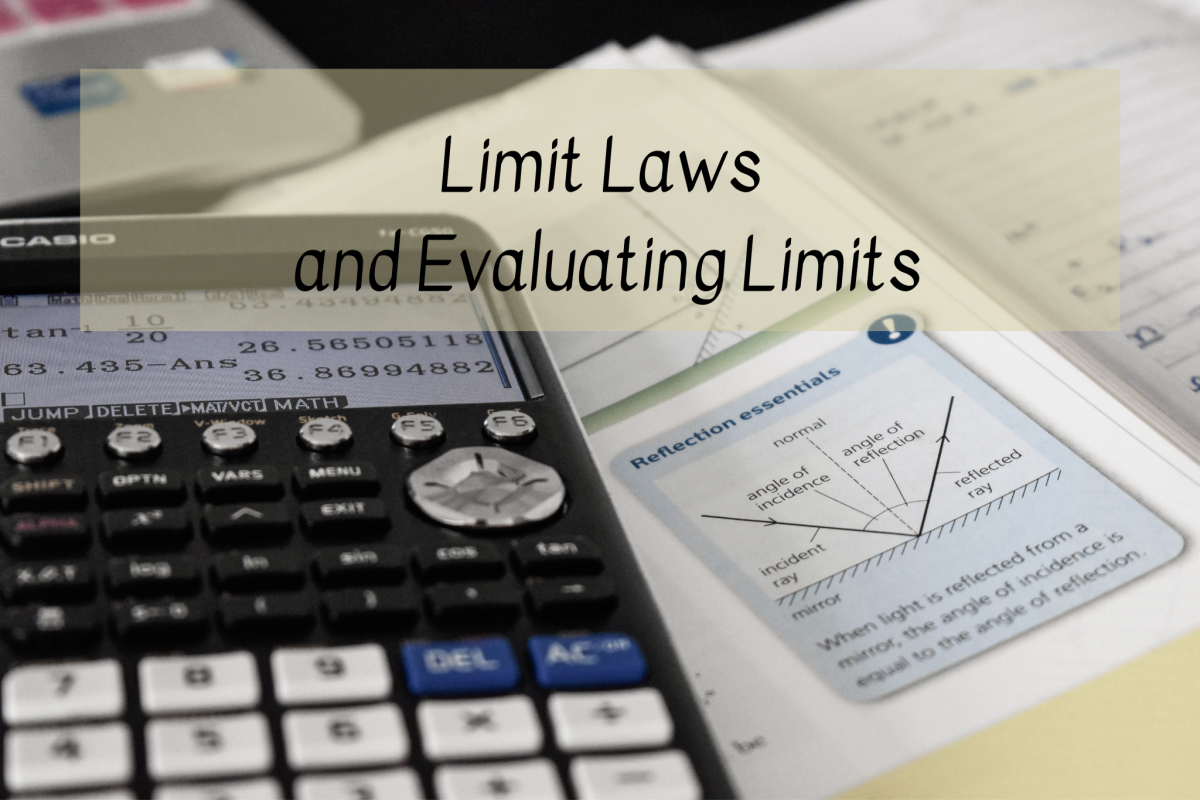Learn how to evaluate limits Photo by Anoushka P on Unsplash

## What Are Limit Laws?

Limit laws are individual properties of limits used to evaluate limits of different functions without going through a detailed process. Limit laws are useful in calculating limits because calculators and graphs do not always lead to the correct answer. In short, limit laws are formulas that help in calculating limits precisely.

For the following limit laws, assume c is a constant and the limit of f(x) and g(x) exist, where x is not equal to an over some open interval containing a.

### Constant Law for Limits

The limit of a constant function c is equal to the constant.

limx →a c = c

### Sum Law for Limits

The limit of a sum of two functions is equal to the sum of the limits.

limx→a [f(x) + g(x)] = limx→a f(x) + limx→a g(x)

### Difference Law for Limits

The limit of a difference of two functions is equal to the difference of the limits.

limx→a [f(x) − g(x)] = limx→a f(x) − limx→a g(x)

### Constant Multiple Law/Constant Coefficient Law for Limit

The limit of a constant multiplied by a function is equal to the constant times the limit of the function.

limx→a [c f(x)] = c limx→a f(x)

### Product Law/Multiplication Law for Limits

The limit of a product is equal to the product of the limits.

limx→a [f(x)g(x)] = limx→a f(x) × limx→a g(x)

### Quotient Law for Limits

The limit of a quotient is equal to the quotient of the numerator and denominator's limits, provided that the denominator's limit is not 0.

limx→a [f(x)/g(x)] = limx→a f(x) / limx→a g(x)

Scroll to Continue

## Read More From Owlcation

### Identity Law for Limits

The limit of a linear function is equal to the number x is approaching.

limx→a x = a

### Power Law for Limits

The limit of the power of a function is the power of the limit of the function.

limx→a [f(x)]n = [limx→a f(x)]n

### Power Special Limit Law

The limit of x power is a power when x approaches a.

limx→a xn = an

### Root Law for Limits

Where n is a positive integer & if n is even, we assume that limx→a f(x) > 0.

limx→an√f(x) = n√limx→a f(x)

### Root Special Limit Law

Where n is a positive integer & if n is even, we assume that a > 0.

limx→an√x = n√a

### Composition Law for Limits

Suppose limx→a g(x) = M, where M is a constant. Also, suppose f is continuous at M. Then,

limx→a f(g(x)) = f(limx→a (g(x)) = f(M)

### Inequality Law for Limits

Suppose f(x) ≥ g(x) for all x near x = a. Then,

limx→a f(x) ≥ limx→a g(x)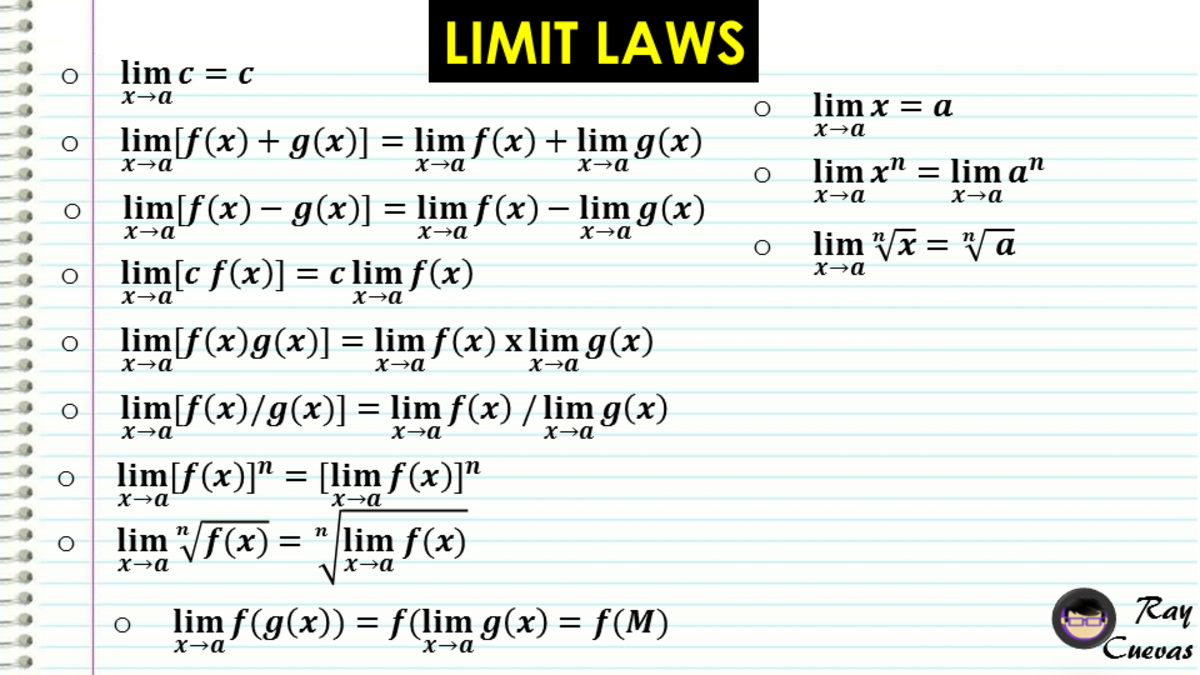Limit Laws in CalculusJohn Ray Cuevas

## Example 1

### Evaluating the Limit of a Constant

Evaluate the limit limx→7 9.

### Solution

Solve by applying the Constant Law for Limits. Since y is always equal to k, it does not matter what x approaches.

limx→7 9 = 9

The limit of 9 as x approaches seven is 9.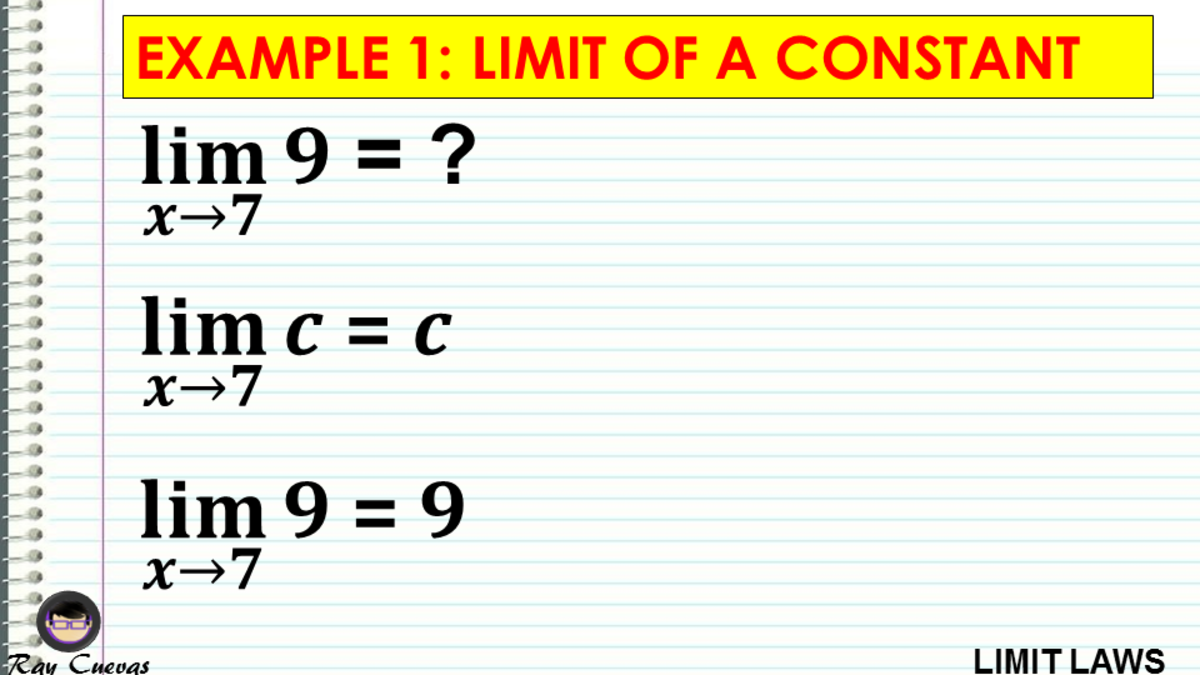Example 1: Evaluating the Limit of a ConstantJohn Ray Cuevas

## Example 2: Evaluating the Limit of a Sum

Solve for the limit of limx→8 (x+10).

Solution

When solving for the limit of an addition, take each term's limit individually, then add the results. It is not limited to two functions only. It will work no matter how many functions are separated by the plus (+) sign. In this case, get the limit of x and separately solve for the limit of the constant 10.

limx→8 (x+10) = limx→8 (x) + limx→8 (10)

The first term uses Identity law, while the second term uses the constant law for limits. The limit of x as x approaches eight is 8, while the limit of 10 as x approaches eight is 10.

limx→8 (x+10) = 8+10

limx→8 (x+10) = 18

The limit of x+10 as x approaches eight is18.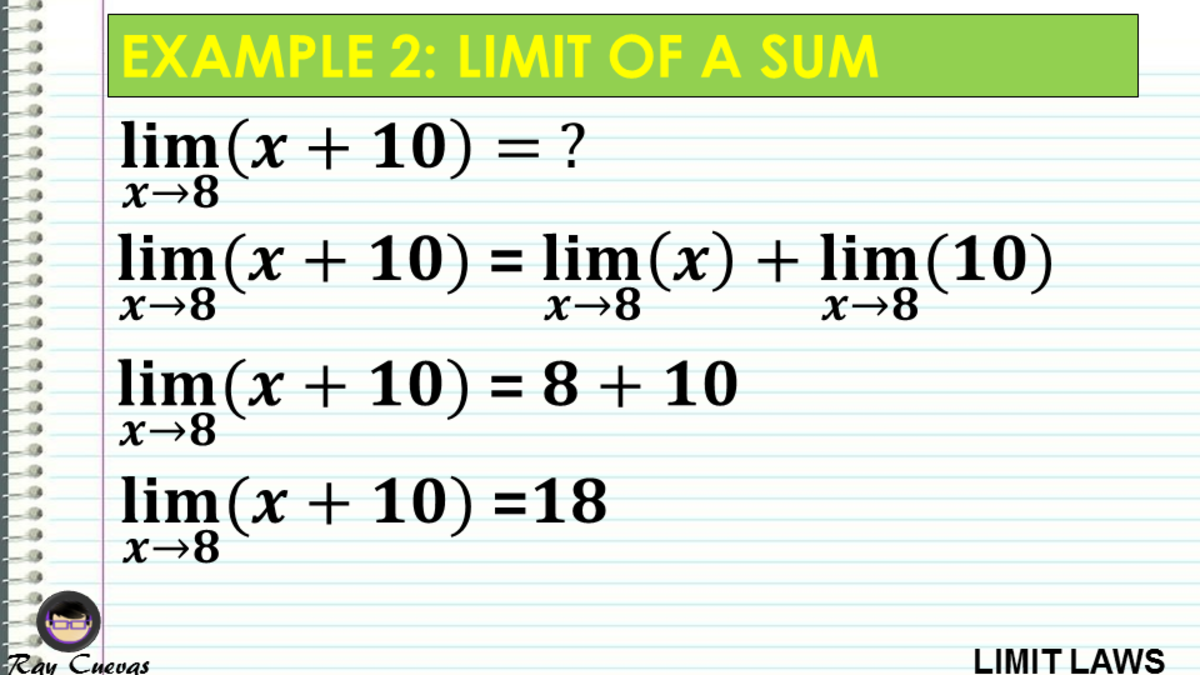Example 2: Evaluating the Limit of a SumJohn Ray Cuevas

## Example 3: Evaluating the Limit of a Difference

Calculate the limit of limx→12 (x−8).

Solution

When taking the limit of a difference, take the limit of each term individually, and then subtract the results. It is not limited to two functions only. It will work no matter how many functions are separated by the minus (-) sign. In this case, get the limit of x and separately solve the constant 8.

limx→12 (x−8) = limx→12 (x) + limx→12 (8)

The first term uses Identity law, while the second term uses the constant law for limits. The limit of x as x approaches 12 is 12, while the limit of 8 as x approaches 12 is 8.

limx→12 (x−8) = 12−8

limx→12 (x−8) = 4

The limit of x-8 as x approaches 12 is 4.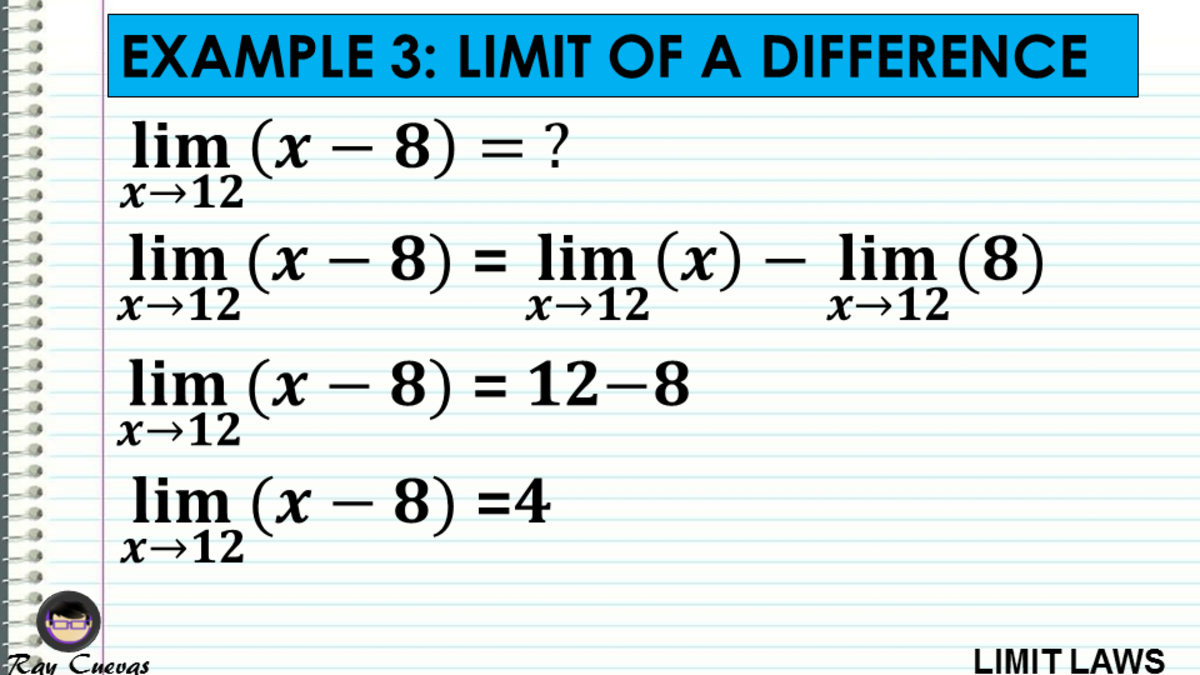Example 3: Evaluating the Limit of a DifferenceJohn Ray Cuevas

## Example 4: Evaluating the Limit of a Constant Times the Function

Evaluate the limit limx→5 (10x).

Solution

If solving limits of a function that has a coefficient, take the function's limit first, and then multiply the limit to the coefficient.

limx→5 (10x) = 10 limx→5 (x)

limx→5 (10x) = 10(5)

limx→5 (10x) = 50

The limit of 10x as x approaches five is 50.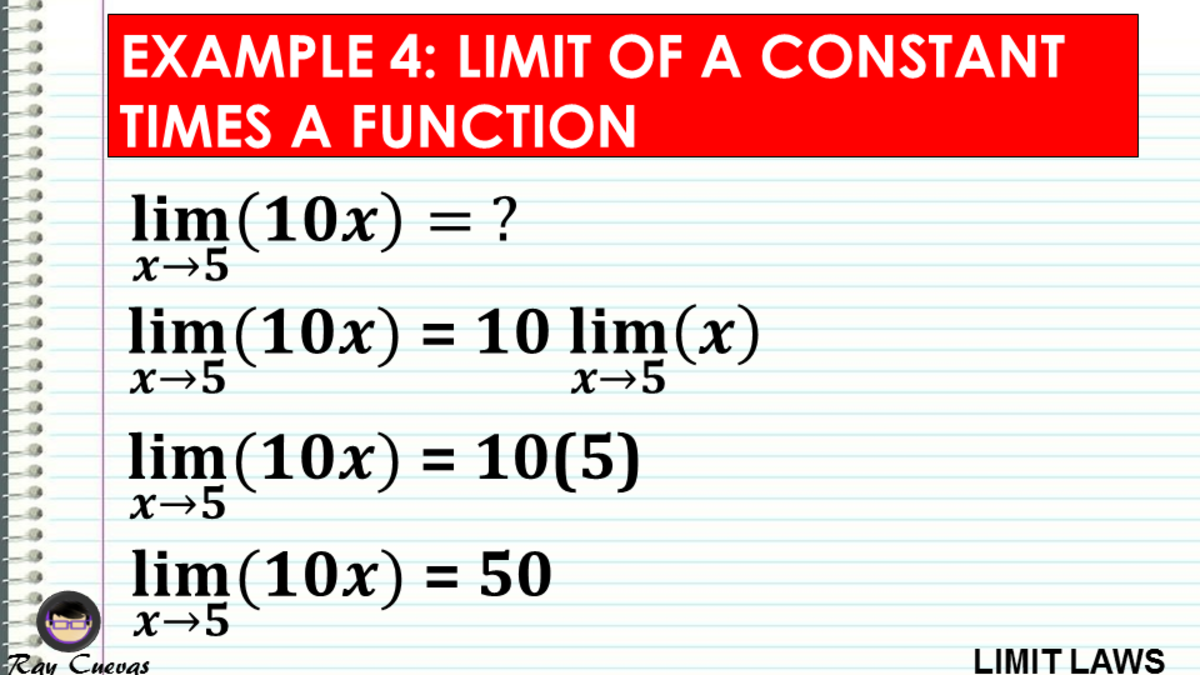Example 4: Evaluating the Limit of a Constant Times the FunctionJohn Ray Cuevas

## Example 5: Evaluating the Limit of a Product

Evaluate the limit limx→2 (5x3).

Solution

This function involves the product of three factors. First, take the limit of each factor, and multiply the results with coefficient 5. Apply both the multiplication law and identity law for limits.

limx→2 (5x3) = 5 limx→2 (x) × limx→2 (x) × limx→2 (x)

Apply the coefficient law for limits.

limx→2 (5x3) = 5(2)(2)(2)

limx→2 (5x3) = 40

The limit of 5x3 as x approaches two is 40.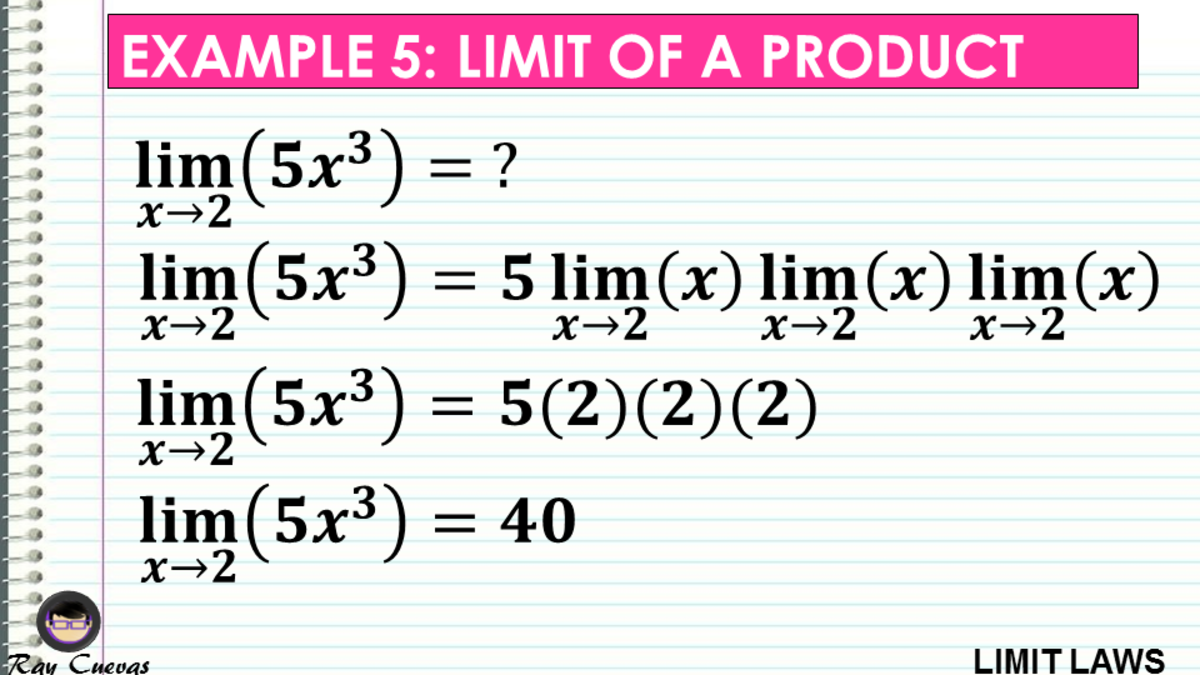Example 5: Evaluating the Limit of a ProductJohn Ray Cuevas

## Example 6: Evaluating the Limit of a Quotient

Evaluate the limit limx→1 [(3x)/(x+5)].

Solution

Using the division law for limits, find the numerator's limit and the denominator separately. Make sure that the value of the denominator will not result in 0.

limx→1 [(3x)/(x+5)] = [limx→1 (3x)] / [limx→1 (x+5)]

Apply the constant-coefficient law on the numerator.

limx→1 [(3x)/(x+5)] = 3 [limx→1 (x)] / [limx→1 (x+5)]

Apply the sum law for limits on the denominator.

limx→1 [(3x)/(x+5)] = [3 limx→1 (x)] / [limx→1 (x) + limx→1 (5)]

Apply the identity law and constant law for limits.

limx→1 [(3x)/(x+5)] = 3(1)/(1+5)

limx→1 [(3x)/(x+5)] = 1/2

The limit of (3x)/(x+5) as x approaches one is 1/2.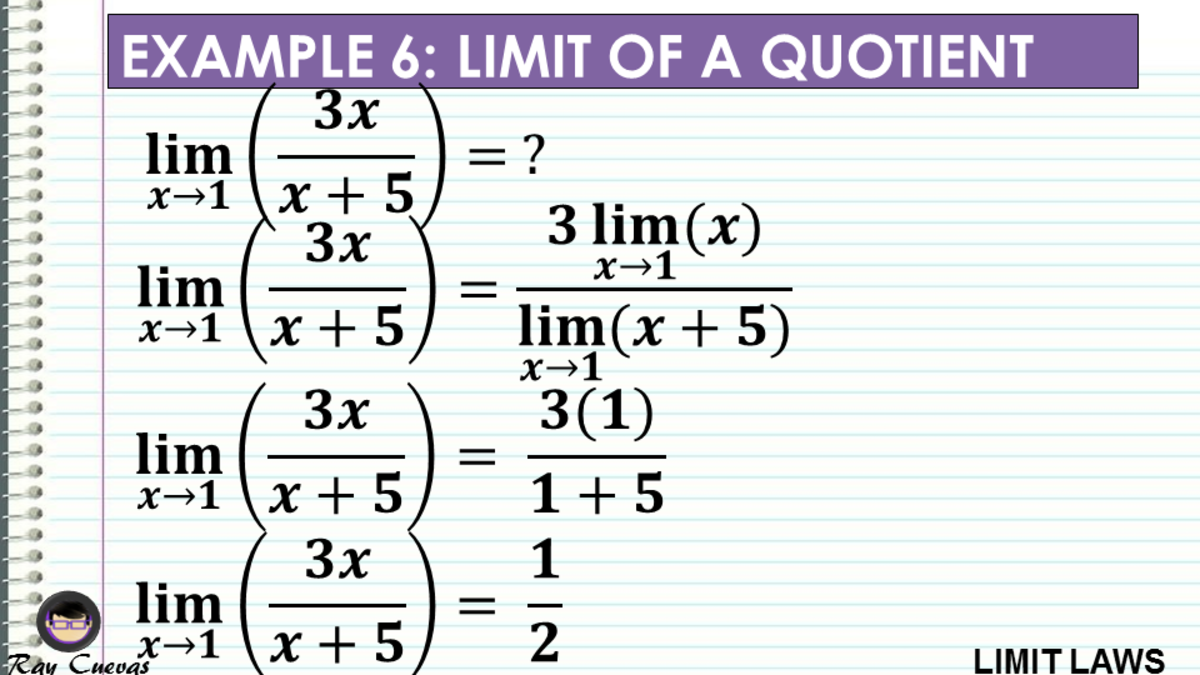Example 6: Evaluating the Limit of a QuotientJohn Ray Cuevas

## Example 7: Evaluating the Limit of a Linear Function

Calculate the limit limx→3 (5x − 2).

Solution

Solving the limit of a linear function applies different laws of limits. To start, apply the subtraction law for limits.

limx→3 (5x − 2) = limx→3 (5x) − limx→3 (2)

Apply the constant-coefficient law in the first term.

limx→3 (5x − 2) = 5 limx→3 (x) − limx→3 (2)

Apply identity law and constant law for limits.

limx→3 (5x − 2) = 5(3) − 2

limx→3 (5x − 2) = 13

The limit of 5x-2 as x approaches three is 13.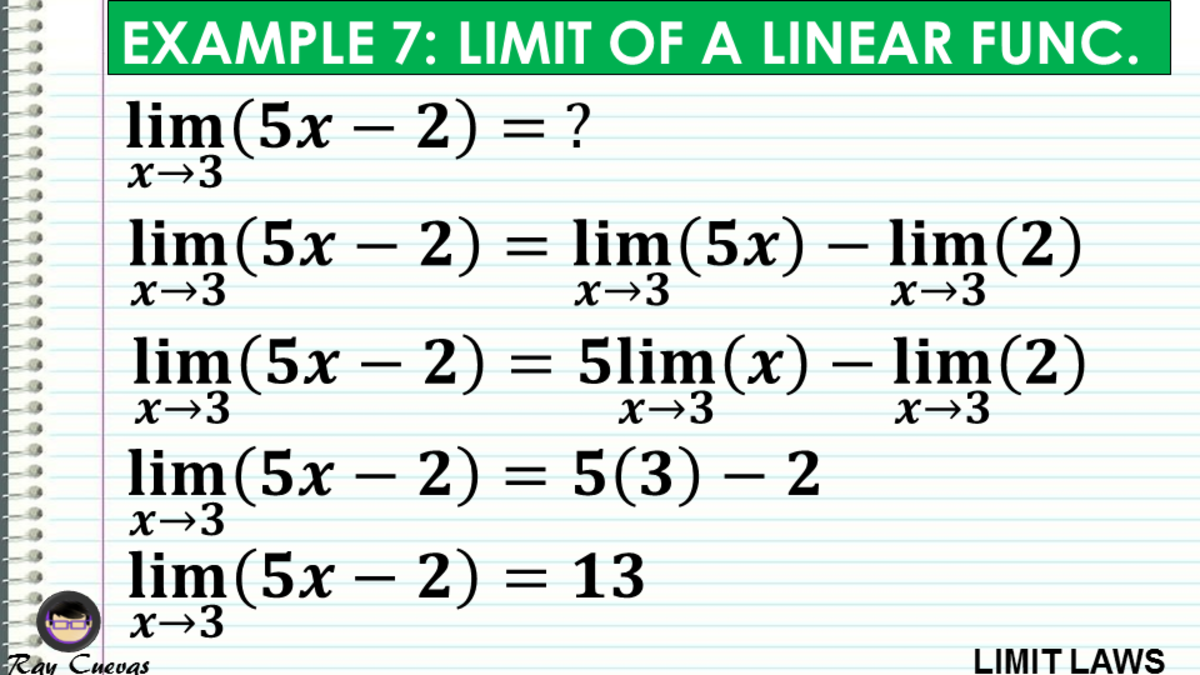Example 7: Evaluating the Limit of a Linear FunctionJohn Ray Cuevas

## Example 8: Evaluating the Limit of the Power of a Function

Evaluate the limit of the function limx→5 (x + 1)2.

Solution

When taking limits with exponents, limit the function first, and then raise to the exponent. Firstly, apply the power law.

limx→5 (x + 1)2 = (limx→5 (x + 1))2

Apply the sum law for limits.

limx→5 (x + 1)2 = [limx→5 (x) + limx→5 (1)]2

Apply the identity and constant laws for limits.

limx→5 (x + 1)2 = (5 + 1)2

limx→5 (x + 1)2 = 36

The limit of (x+1)2 as x approaches five is 36.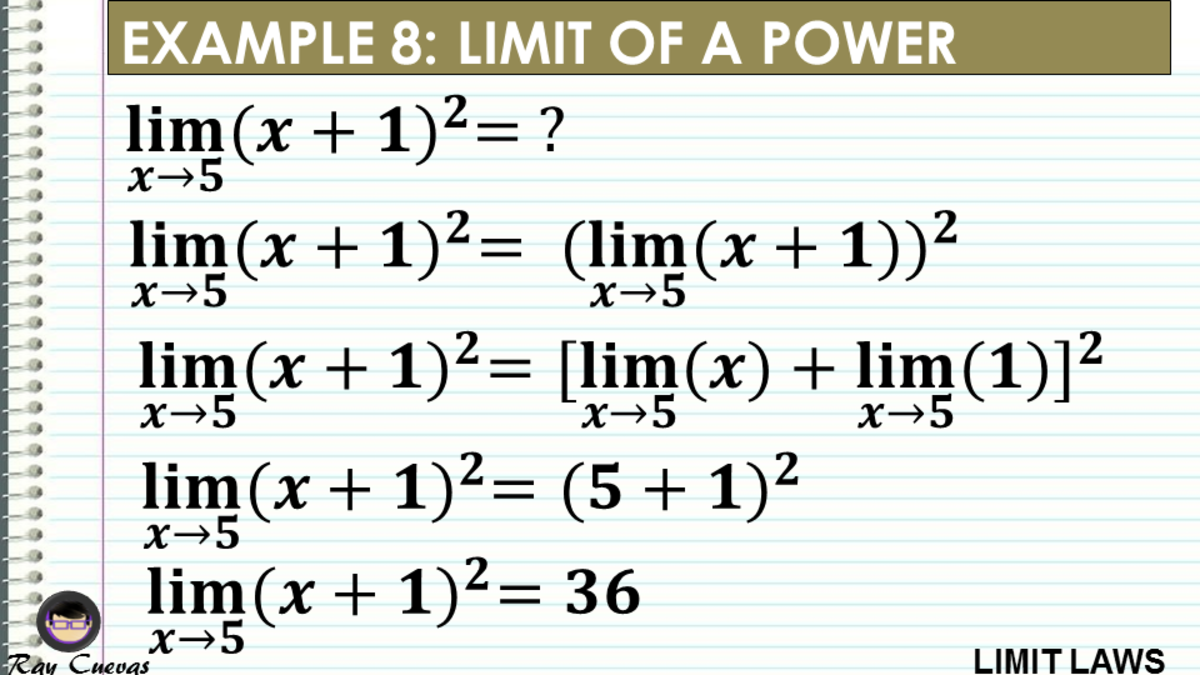Example 8: Evaluating the Limit of the Power of a FunctionJohn Ray Cuevas

## Example 9: Evaluating the Limit of Root of a Function

Solve for the limit of limx→2 √(x+14).

Solution

In solving for the limit of root functions, find first the limit of the function side the root, and then apply the root.

limx→2 √x+14 = √[limx→2 (x + 14)]

Apply the sum law for limits.

limx→2 √x+14 = √[limx→2 (x) + limx→2 (14)]

Apply identity and constant laws for limits.

limx→2 √ (x+14) = √(16)

limx→2 √(x+14) = 4

The limit of √(x+14) as x approaches two is 4.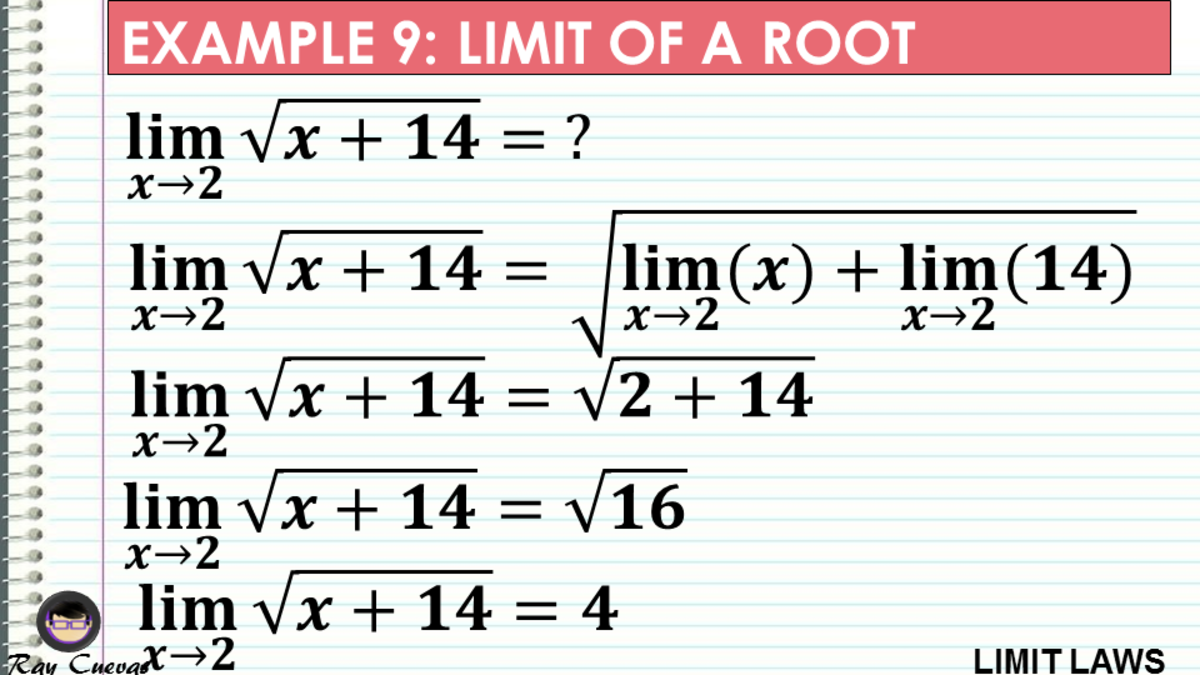Example 9: Evaluating the Limit of Root of a FunctionJohn Ray Cuevas

## Example 10: Evaluating the Limit of Composition Functions

Evaluate the limit of the composition function limx→π [cos(x)].

Solution

Apply the composition law for limits.

limx→π [cos(x)] = cos (limx→π (x))

Apply the identity law for limits.

limx→π cos(x) = cos(π)

limx→π cos(x) = −1

The limit of cos(x) as x approaches π is -1.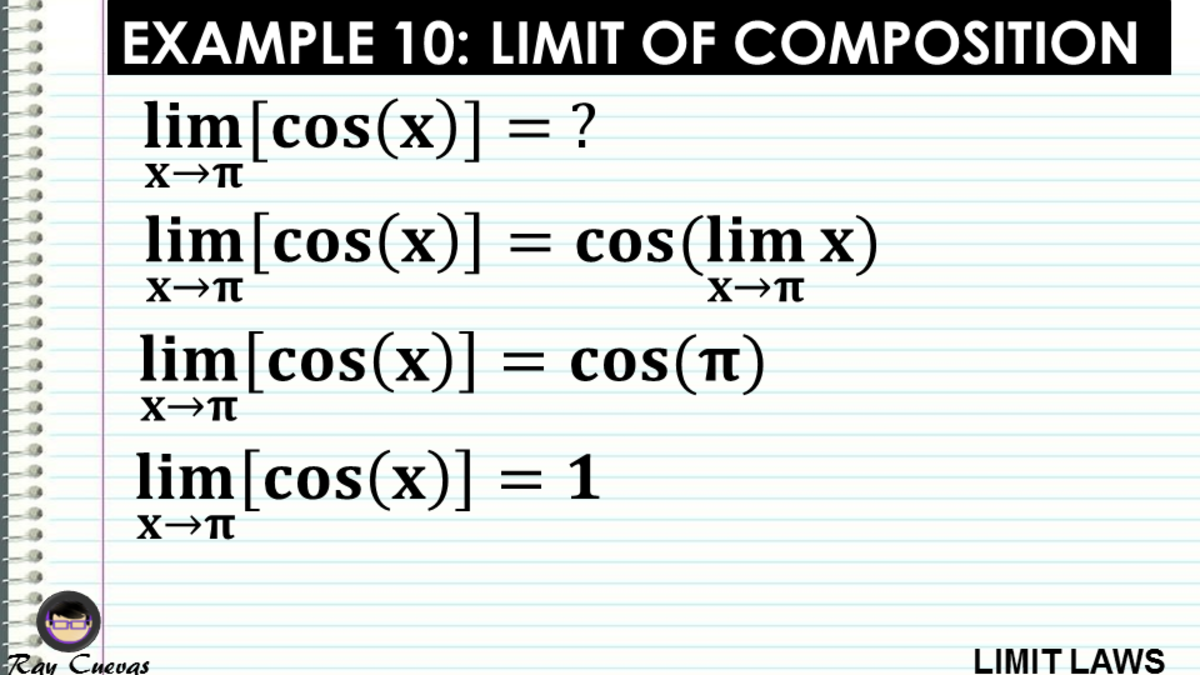Example 10: Evaluating the Limit of Composition FunctionsJohn Ray Cuevas

## Example 11: Evaluating the Limit of Functions

Evaluate the limit of the function limx→5 2x2−3x+4.

Solution

Apply the addition and difference law for limits.

limx→5 (2x2 − 3x + 4) = limx→5 (2x2) − limx→5 (3x) + limx→5 (4)

Apply the constant-coefficient law.

limx→5 2x2 − 3x + 4 = 2 limx→5 (x2) – 3 limx→5 (x) + limx→5 (4)

Apply the power rule, constant rule, and identity rules for limits.

limx→5 2x2 − 3x + 4 = 2(52) − 3(5) + 4

limx→5 2x2 − 3x + 4 = 39